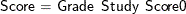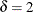# The MI Procedure

### Example 63.17 Adjusting Imputed Classification Levels in Sensitivity Analysis

This example illustrates the pattern-mixture model approach to multiple imputation under the MNAR assumption by adjusting imputed classification levels.

Carpenter and Kenward (2013, pp. 240–241) describe an implementation of sensitivity analysis that adjusts an imputed missing covariate, where the covariate is a nominal classification variable.

Suppose a high school class is conducting a study to analyze the effects of an extra web-based study class and grade level on the improvement of test scores. The regression model that is used for the study iswhere Grade is the grade level (with the values 6 to 8), Study is an indicator variable (with the values 1 for "completes the study class" and 0 for "does not complete the study class"), Score0 is the current test score, and Score is the test score for the subsequent test.

Also suppose that Study, Score0, and Score are fully observed and the classification variable Grade contains missing grade levels. Output 63.17.1 lists the first 10 observations in the data set Mono2.

Output 63.17.1: Student Test Data

 First 10 Obs in the Student Test Data

Obs Grade Score0 Score Study
1 6 64.4898 68.8210 1
2 6 72.0700 76.5328 1
3 6 65.7766 75.5567 1
4 . 70.2853 76.0180 1
5 6 74.3388 80.0617 1
6 6 70.2207 76.1606 1
7 6 68.6904 77.9770 1
8 . 72.6758 79.6895 1
9 6 64.8939 69.3889 1
10 6 66.6038 72.7793 1

The following statements use the MONOTONE and MNAR statements to impute missing values for Grade under the MNAR assumption:

proc mi data=Mono2 seed=34857 nimpute=10 out=outex17;
class Study Grade;
monotone logistic (Grade / link=glogit);
mnar adjust( Grade (event='6') /shift=2);
var Study Score0 Score Grade;
run;


The LINK=GLOGIT suboption specifies that the generalized logit function be used in fitting the logistic model for Grade. The ADJUST option specifies a shift parameterthat is applied to the generalized logit model function values for the response level GRADE=6. This assumes that students who have a missing grade level are more likely to be students in grade 6.

The "Model Information" table  in Output 63.17.2 describes the method that is used in the multiple imputation process.

Output 63.17.2: Model Information

The MI Procedure

Model Information
Data Set WORK.MONO2
Method Monotone
Number of Imputations 10
Seed for random number generator 34857

The "Monotone Model Specification"  table in Output 63.17.3 describes methods and imputed variables in the imputation model. The MI procedure uses the logistic regression method (generalized logit model) to impute the variable Grade.

Output 63.17.3: Monotone Model Specification

Monotone Model Specification
Method Imputed Variables
Regression Score0 Score
Logistic Regression Grade

The "Missing Data Patterns"  table in Output 63.17.4 lists distinct missing data patterns and their corresponding frequencies and percentages.

Output 63.17.4: Missing Data Patterns

Missing Data Patterns
Group Study Score0 Score Grade Freq Percent Group Means
Score0 Score
1 X X X X 128 85.33 70.418230 74.469573
2 X X X . 22 14.67 69.338503 73.666293

The "MNAR Adjustments to Imputed Values"  table in Output 63.17.5 lists the adjustment parameter for the 10 imputations.

Output 63.17.5: MNAR Adjustments to Imputed Values

MNAR Adjustments to
Imputed Values
Imputed
Variable
Event Shift
Grade 6 2.0000

The following statements list the first 10 observations of the data set Outex17 in Output 63.17.6:

proc print data=outex17(obs=10);
var _Imputation_ Grade Study Score0 Score;
title 'First 10 Observations of the Imputed Student Test Data Set';
run;


Output 63.17.6: Imputed Data Set

 First 10 Observations of the Imputed Student Test Data Set

Obs _Imputation_ Grade Study Score0 Score
1 1 6 1 64.4898 68.8210
2 1 6 1 72.0700 76.5328
3 1 6 1 65.7766 75.5567
4 1 6 1 70.2853 76.0180
5 1 6 1 74.3388 80.0617
6 1 6 1 70.2207 76.1606
7 1 6 1 68.6904 77.9770
8 1 6 1 72.6758 79.6895
9 1 6 1 64.8939 69.3889
10 1 6 1 66.6038 72.7793